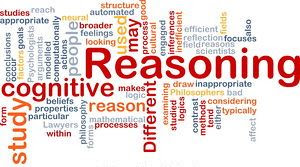# Reasoning for Bank Exams - IBPS POReasoning for IBPS PO
Welcome to Mentor for Bank Exams. Here I am posting some important Reasoning Practice Questions with explanation for Upcoming IBPS PO/RRB Exams. Candidates those who are preparing for those exams can use this practice questions.
Directions (1-6) : Study the following information carefully and answer the questions given below.
Twelve friends P, Q, R, S, T, U, V, W, X, Y, Z and M are sitting in a row facing east. Y sits third to the left of T, who sits sixth to the right of P. V sits fourth to the right of M, who is not an immediate neighbour of R. Q sits fifth from the right end and W sits second to the left of S. T is not an immediate neighbour of X or U, but sits second to the right of Q. There are two friends between R and Z. Z is not an immediate neighbour of Y.
1. What is the position of U with respect to W?
(1) Third to the right
(2) Second to the left
(3) Seventh to the left
(4) Can't be determined
(5) None of these
2. If Z is an immediate neighbour of X, then who among the following is on the immediate right of S?
(1) U
(2) T
(3) M
(4) P
(5) None of these
3. How many persons are there between V and T?
(1) Two
(2) Three
(3) four
(4) five
(5) None of these
4. If U is immediate neighbour of M, then who among the following sit on the extreme ends of the line?
(1) ZS
(2) MX
(3) WU
(4) XU
(5) None of these
5. Who among the following sits third to the right of the eighth from the right end?
(1) Q
(2) W
(3) T
(4) U
(5) None of these
6. Four of the following five are alike in a certain way and hence form a group. Which is the one that does not belong to that group?
(1) RP
(2) QR
(3) YW
(4) TQ
(5) ZM
Directions (7 – 10) : Study the following information carefully and answer the questions given below.
Six students A, B, C, D, E and F obtained different marks in an examination. A obtained more marks than only two students. B obtained more marks than D but not the highest. F obtained more marks than only C. The one who obtained the second highest marks obtained 280. The one who obtained the second lowest marks obtained 190 marks.
7. If the total marks of the examination is 500, then who among the following possibly obtained 60% marks?
(1) D
(2) E
(3) Either A or C
(4) Either D or E
(5) None of these
8. If A Obtained 200 marks, then who among the following possibly obtained 245 marks?
(1) D
(2) B
(3) E
(4) F
(5) None of these
9. Who among the following obtained the lowest marks?
(1) F
(2) E
(3) C
(4) B
(5) None of these
10. Who among the following obtained the lowest marks?
(1) F
(2) E
(3) C
(4) B
(5) None of these
1) 4   2) 1   3) 3   4) 2   5) 1   6) 3  7) 2   8) 1   9) 3   10) 2
Solutions:
(1 – 6):

1. (4)
2. (1)
3. (3)
4. (2)
5. (1) Third to the right of eight from the right end = (8 – 3 = 5th) from the right end, ie Q.
6. (3)
7. (2) 60% of 500 = 300
Hence the possible marks 300 were obtained by E.
8. (1)
9. (3)
10. (2)